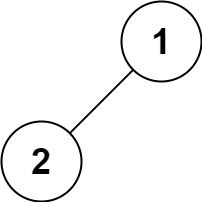| English | 简体中文 |

# 144. Binary Tree Preorder Traversal

## Description

Given the root of a binary tree, return the preorder traversal of its nodes' values.

Example 1:Input: root = [1,null,2,3]
Output: [1,2,3]


Example 2:

Input: root = []
Output: []


Example 3:

Input: root = 
Output: 


Example 4:Input: root = [1,2]
Output: [1,2]


Example 5:Input: root = [1,null,2]
Output: [1,2]


Constraints:

• The number of nodes in the tree is in the range [0, 100].
• -100 <= Node.val <= 100